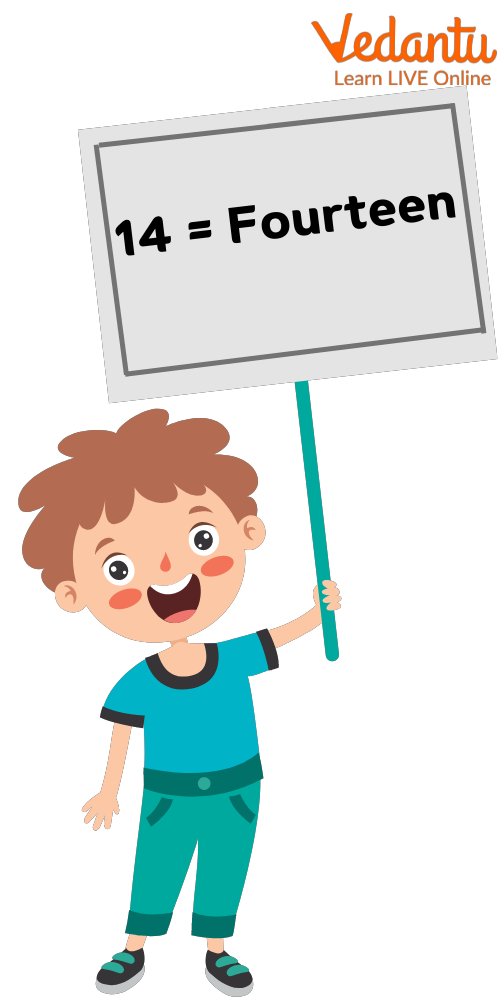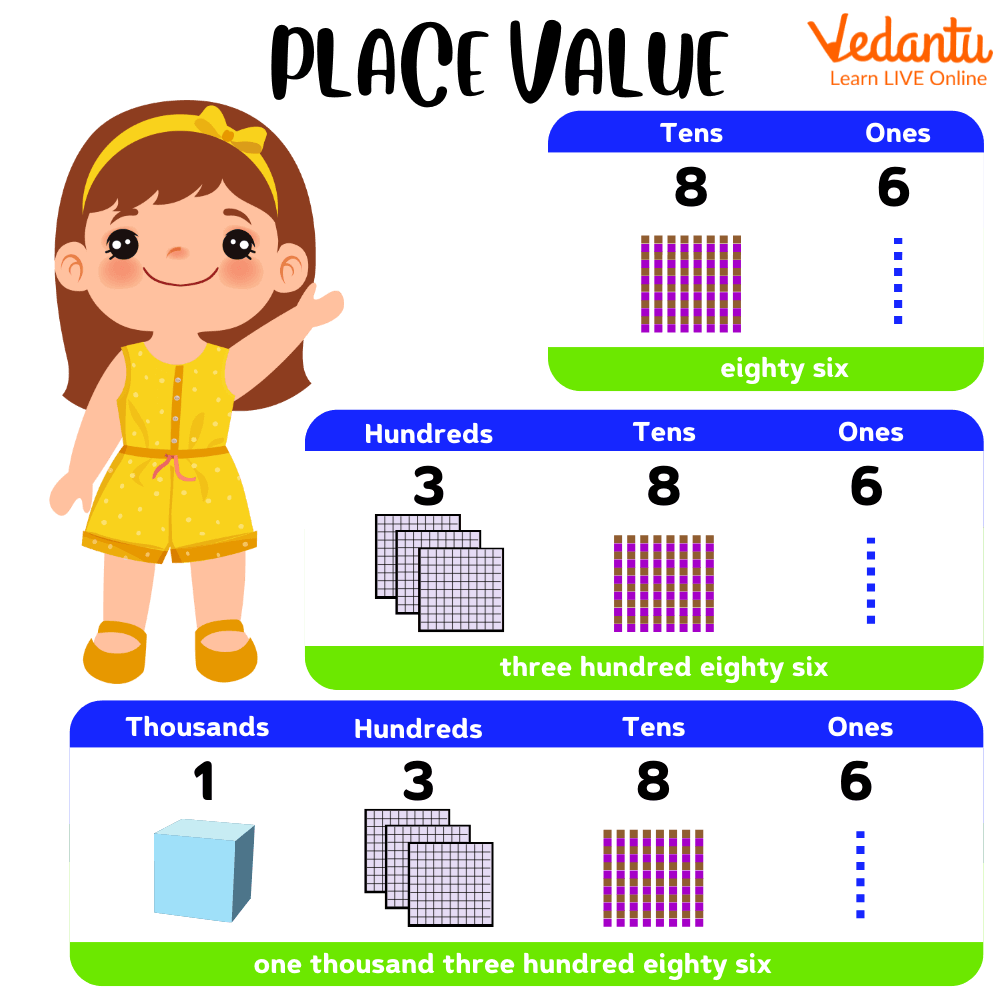Courses
Courses for Kids
Free study material
Free LIVE classes
More

# Writing Number 14 in Words for Kids## An Introduction to Numbers

Have you ever wondered how many stars are there in the sky or how many trees are there in the garden? Well, every child wonders about such a question although one can not exactly answer the question in terms of counting. But there are certain things that can be counted. Quantifying things is one of the important functions of numbers. As kids grow older they start to get curious about nature and things around them, it then really becomes interesting to teach kids about numbers and their uses. The introduction to numbers is the very basic introduction of mathematics to kids.

In this article, we will focus on teaching kids about the fourteenth number in the number system. We aim to teach kids how to write 14 in words and some interesting facts related to the number 14.

## What is a Number?

Before discussing how to write the number 14 in words, let us quickly revise about what a number is. The number can be defined as a mathematical value that can be used for quantifying things which can include measuring, labelling and counting them. Numbers are also used for performing various arithmetic operations. Natural numbers, whole numbers, rational and irrational numbers are classified into the various types of numbers.

## How to Write 14 in Words?

Since we have learnt about what a number is, let us look into how to write 14 numbers in words.

14 in words is written as fourteen.Representing the fourteenth number written in words

## How is the Number Fourteen Written in the Place Value Chart?

Let us first understand what a place value chart is? In Mathematics, place value is defined as the value of each digit in a number that is based on its order. The place value of a number starts with a unit, it is also known as one's place. Ones, tens, hundreds, thousands, ten thousand, and so on are the order of place value of digits in a number from right to left. Let us look at how the fourteen number is written in the place value chart.

 Tens Ones 4 1

The place value chart is read from ones to tens so the 1 in fourteen is at unit place whereas 4 in fourteen is at tens place. The place value of 1 in 14 is a unit but if we consider the palace value of 1 in 2120 is hundred. We can understand the concept of place value by the example mentioned below.Illustrating the place value chart

## Fun Facts About Number Fourteen

• Fourteen is a composite number

• Fourteen is an even number

• Fourteen is not categorised as a prime number.

• The square root of fourteen is 3.741657

In the conclusion of the article, we have learnt to write the number 14 in words, we have also learnt about the definition of the number and the place value chart for the number. We hope to have helped kids build their curiosity in Mathematics to learn more about numbers. Mastering the number and the use of a place value chart for numbers can help kids excel in their grades as well create a strong base for the complex concepts that will be taught in the future grades.

Last updated date: 24th Sep 2023
Total views: 137.7k
Views today: 3.37k

## FAQs on Writing Number 14 in Words for Kids

1. Why is 14 not a prime number?

Prime numbers are defined as the number that can only have two factors, in simpler terms, they are only divisible by 1 and themselves. In the case of 14, it is divisible by 1, 2, 7, 14; hence is not classified as a prime number.

2. Is 14 classified as a whole number?

The set of all the natural numbers along with zero is classified as a whole number. Since 14 is a natural number, it is also classified as a whole number.

3. Why is 14 termed as a composite number?

Composite numbers are defined as numbers that have more than two factors, in simpler terms, they are divisible by more than 2 numbers. In the case of 14, it is divisible by 1, 2, 7, and 14; thus it has four factors. Hence, it is classified as a composite number.